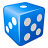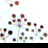# Converting points to Polygons of a defined size

1792
2
04-21-2014 02:13 AMNew Contributor II
Hi Guys ,

i am not sure if this is in the correct place. i hope so.

What i would like to do is take some point data and convert it into polygons .

i would like each of my polygons to be 172 metres long and 124 metres wide i was wondering if there is some where i can do this in the conversion procress. i have look at certain tools but do not see an element for inputting this.

Although i am very new , so maybe i m missing something

Does any one have some tips

Thanks Jack
Tags (3)
2 RepliesRegular Contributor II
It is likely that it would be simplist to
use a search cursor to get the location of each point.
Then construct calculate the location of the other polygon corners based on offsets from that point.
Finally, use an insert cursor to add the constructed polygon to a new polygon feature class.

Writing geometries is a useful phrase to look up in the Help.Frequent Contributor II
There are a number of ways I would approach this.  Since each of your polygons is the same size IF you don't have a lot of points to do this to.  (by not a lot I mean less than 200 or so) the most simple method may be to simply create one polygon of the correct dimensions and the copy paste that polygon and move them such that the points are the center of the polygon.

Now I have a way that is a lot more involved but would allow you to do it to thousands at a time.

The idea here is to create the polylines of the polygon that each have a unique identifier from the point feature.

Calculate the XY of each point.  Create 4 new sets of XY to represent the 4 corners of the polygon.  Call them something you can remember and that makes sense.  Something light URXY, (upper right XY) ULXY, LLXY and LRXY

Manually create the first polygon.  Then generate a centroid.  Using the measurement tool in the same units as your projection (i.e. meters, feet etc...) The distance to the North edge of the polygon and to the east edge of the polygon

IF you want to be as accurate as possible use the COGO tool to generate straight lines to the edges and then trim them to the edge and record the distances.

For that matter generate the polygon using the COGO tool to make a polygon the exact size you want.

Now your X,Y may look like this   10000, 10000 in meters (I am using simple numbers for the example).  For the upper right you subtract 124 from the X and Add 172 to the Y giving a new corner XY of 9876,10172.  Do the rest for the other 4.  Draw it out on paper first to make sure you are adding and subtracting the correct way for each corner.

Now you will do the next step 4 times.  Use the XY to polyline tool and generate lines from URXY to ULXY, then URXY to LLXY, and LLXY to LRXY, and LRXY to URXY.

The XY to polyline will allow you to save a single attribute to the new feature.  Use the polyline to polygon tool to create polygons

You may need to export the Point feature to a .csv layer to use the xy to poyline tool

I may not have explained this properly but doing it this way should allow you to create thousands of polygons in about 30 to 45 minutes or less.

Hi Guys ,

i am not sure if this is in the correct place. i hope so.

What i would like to do is take some point data and convert it into polygons .

i would like each of my polygons to be 172 metres long and 124 metres wide i was wondering if there is some where i can do this in the conversion procress. i have look at certain tools but do not see an element for inputting this.

Although i am very new , so maybe i m missing something

Does any one have some tips

Thanks Jack